DAY 26
0
Modern Web

處理資料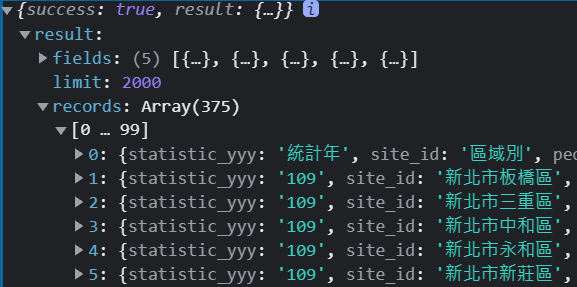使用d3.group結合array.slice群組化資料

d3.json("populationDensity.json")
.then(function (data) {
console.log(data);
let districtData = d3.group(data.result.records,d=>{
return d.site_id.slice(0, 3);
})
console.log(districtData);
}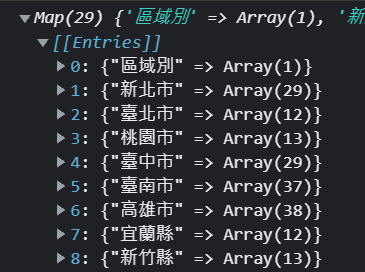用Array.from將Map物件轉換成Array

const districtAry = Array.from(districtData);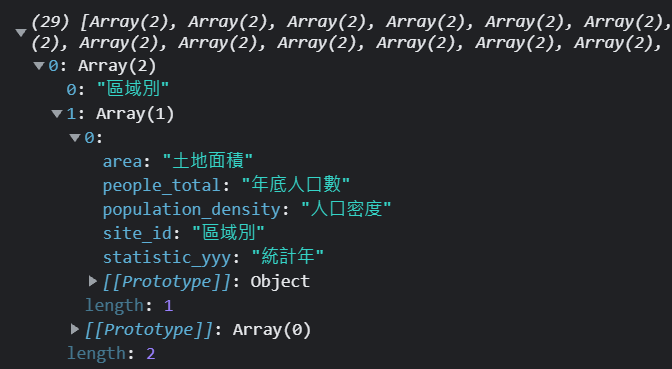清理資料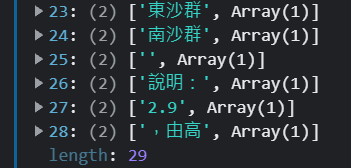districtAry.splice(-6);
districtAry.shift();

增加一個元素為該縣市的人口數

districtAry.forEach(function (el) {
el.push(d3.sum(el, (d) => Number(d.people_total)));
});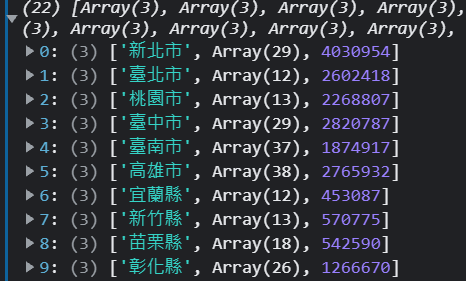增加一個元素寫入是否為直轄市

let municipality = ["臺北市","新北市","臺南市","高雄市","桃園市","臺中市"];
districtAry.forEach((districtAryEl) => {
for (let i = 0; i < municipality.length; i++) {
if (districtAryEl == municipality[i]) {
districtAryEl = "直轄市";
return;
} else {
districtAryEl = "非直轄市";
}
}
});

處理完畢直轄市、非直轄市、全台人口數加總

const municipalitySumFun = (municipalityStr) =>
d3.sum(districtAry, (d) => {
if (d === municipalityStr) return d;
if (municipalityStr==undefined) return d;
});
let districtArySum = municipalitySumFun();
let municipalitySum = municipalitySumFun("直轄市");
let NotMunicipalitySum = municipalitySumFun("非直轄市");

console.log(districtArySum);
console.log(municipalitySum);
console.log(NotMunicipalitySum);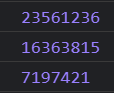arc函式和pie函式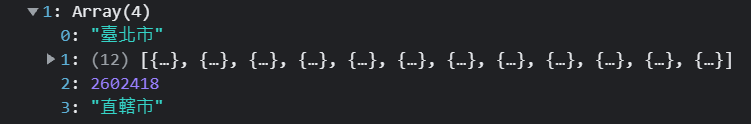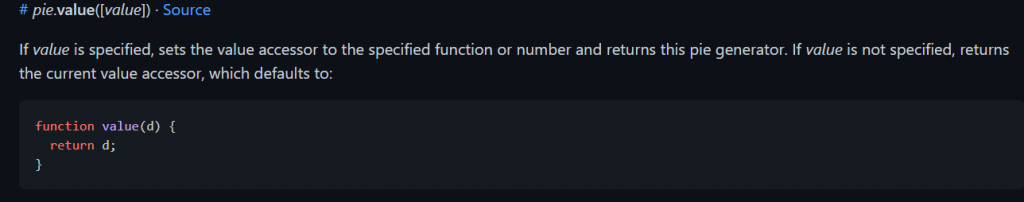d3.pie().value()官方說明

let pie = d3.pie().value(function (d) {
return d;
});
console.log(pie(districtAry));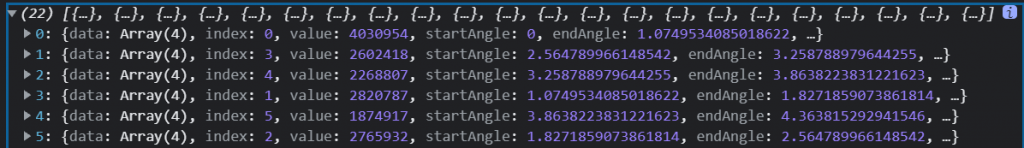開始繪製圖形

let color = d3.scaleOrdinal(d3.schemeTableau10);
g.attr(
"transform",
`translate(\${width / 2}, \${height / 2}) scale(2.5)`
)
g.attr(
"transform",
`translate(\${width / 2}, \${height / 2}) scale(2.5)`
)
g.selectAll("g")
.data(d3.sort(pie(districtAry), (d) => d.index))
.join("g")
.append("path")
.attr("stroke", "white")
.attr("stroke-width", ".25")
.attr("fill", (d, i) => color(d.data))
.attr("d",function(d){
return arc(d);
})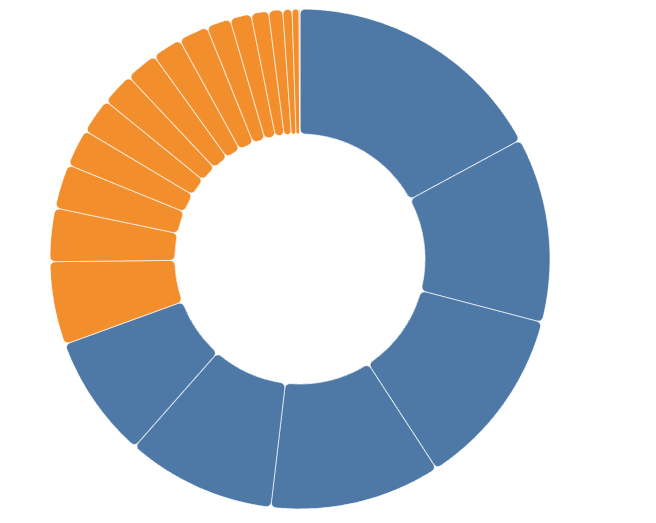添加動畫

g.selectAll("g")
.data(d3.sort(pie(districtAry), (d) => d.index))
//中間省略
//中間省略
//中間省略
.transition()
.ease(d3.easeLinear)
.duration(500)
.delay(function (d, i) {
return i*500;
})
.attr("d",function(d){
return arc(d);
})添加捕間動畫

transition().attrTween()來增加每個扇形區域進行繪製的時候要進行的動畫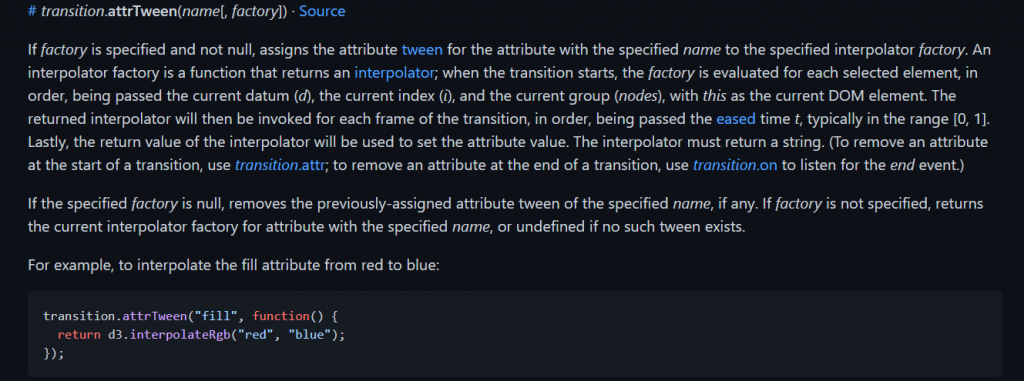//上面省略
.transition()
.ease(d3.easeLinear)
.duration(500)
.delay(function (d, i) {
return i*500;
})
.attrTween("d", function (d) {  //也就是設置每一個d的補間動畫
let i = d3.interpolate(d.startAngle, d.endAngle);
return function (t) {
d.endAngle = i(t);
return arc(d);
};
});

d3.interpolate輸入的參數是0~1計算百分比函式

function Percentage(num, total) {
if (num == 0 || total == 0) {
return 0;
}
return Math.round((num / total) * 10000) / 100.0;
}

添加滑鼠滑入事件

g.selectAll("g")
.on("mouseenter", function (e) {
let appendText = d3
.select(this)
.append("text")
.style("font-size", "10px")
.style("text-anchor", "middle")
.html(d=>{
return `<tspan x="0" y="-.5em">\${d.data}</tspan>
<tspan x="0" y=".5em">\${d.data}人</tspan>
<tspan x="0" y="1.5em">\${Percentage(d.data,districtArySum)}%</tspan>`
})
})
.on("mouseleave", function (e) {
d3.select(this).select("text").remove();
});

svg.append("g").html(`
<text  y="\${height-100}" style="font-size:50; transform:translate(-20%,0)">
<tspan x="\${width/2}">直轄市\${Percentage(municipalitySum,districtArySum)}%</tspan>
<tspan x="\${width/2}"  dx="-0.5em"dy="1em">非直轄市\${Percentage(NotMunicipalitySum,districtArySum)}%</tspan>
</text>
`)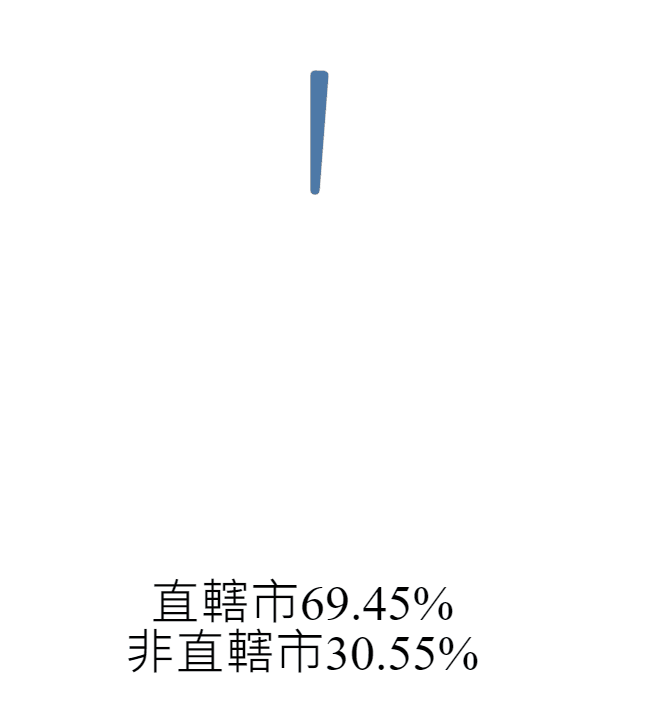gitPage